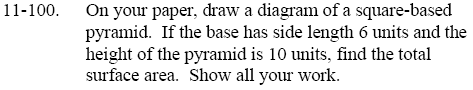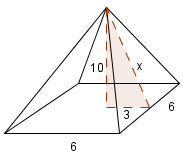### Home > GC > Chapter 11 > Lesson 11.2.3 > Problem11-100

11-100.Use the Pythagorean Theorem to find the slant height of the pyramid, which will be referred to as x.

10² + 3² = x²

$x=\sqrt{109}$Now that you know the slant height you can find the area of the four triangles that make up the pyramid.
Afterwards, add the area of the four triangles to the area of the pyramid's base in order to find the total surface area of the pyramid.

161.28 units²